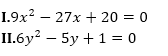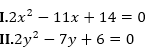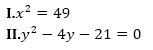We are providing you with the test of the day which will help you in upcoming exams like SBI PO Prelims 2018. From this, you can practice questions with the timer that will help you to improve speed. SBI PO Prelims Exam 2018 is one of the biggest opportunity for you guys to grab on, set you own mark and proceed with full confidence. All the very best for your preparations and All the future exams.

Directions (1-5): In the following questions, the symbols ©, @, \$, % and * are used with the following meanings as illustrated below:
‘P @ Q’ means ‘P is not greater than Q’.
‘P % Q’ means ‘P is not smaller than Q’.
‘P * Q’ means ‘P is neither smaller than nor equal to Q’.
‘P © Q’ means ‘P is neither greater than nor equal to Q’.
‘P \$ Q’ means ‘P is neither greater than nor smaller than Q’.

In each question four statements showing relationship have been given, which are followed by four conclusions I, II, III & IV. Assuming that the given statements are true, find out which conclusion(s) is/are definitely true.

Q1. Statements:
M © D, D * K, K @ R, R * F
Conclusions:
II. D * F
IV. D * R
None is true
Only I is true
Only II is true
Only III is true
Only IV is true
Solution:
Conclusions:
I. F © K ( Not True )
II. D * F ( Not True )
III. M © R ( Not True )
IV. D * R( Not True )

Q2. Statements:
B % K, K \$ T, T * F, H © F
Conclusions:
I. B \$ T
Only either I or II is true
Only III is true
Only IV is true
Only III and IV are true
Only either I or II and III and IV are true
Solution:
Conclusions:
I. B \$ T ( Not True )
II. T © B ( Not True )
III. H © K ( True )
IV. F © B ( True )

Q3. Statements:
W * B, B @ F, F © R, R \$ M
Conclusions:
I. W * F
II. M * B
III. R * B
IV. M * W
Only I and IV are true
Only II and III are true
Only I and III are true
Only II and IV are true
None of these
Solution:
Conclusions:
I. W * F ( Not True )
II. M * B ( True )
III. R * B ( True )
IV. M * W ( Not True )

Q4. Statements:
E @ K, K \$ T, T © N, B % N
Conclusions:
I. T % E
III. B * T
IV. B * E
Only I, II and III are true
Only II, III and IV are true
Only I, III and IV are true
All are true
None of these
Solution:
Conclusions:
I. T % E ( True )
II. K © N ( True )
III. B * T ( True )
IV. B * E ( True )

Q5. Statements:
Z \$ B, B % M, M © F, F @ R
Conclusions:
I. Z * M
II. F * B
III. R * M
IV. M @ Z
Only I and II are true
Only I, III and IV are true
Only III and IV are true
Either I or IV and II are true
None of these
Solution:
Conclusions:
I. Z * M ( Not True )
II. F * B ( Not True )
III. R * M ( True )
IV. M @ Z ( True )

Directions (6-10): In each of the question given below a/an idiom/phrase is given in bold which is then followed by five options which then try to decipher its meaning. Choose the option which gives the correct meaning of the phrases.

Q6. Better late than never
Better to stay healthy than to die
Better to arrive late than to meet with an accident
Better to come late than to die
Better to stay home than to reach late
Better to arrive late than not to come at all
Solution:
Meaning: It’s better to arrive late than not to come at all

Q7. Cut somebody some slack
Not to be so benevolent
Not to be so rude
Not to be so critical
Not to be so happy
Not to be so boring
Solution:
Meaning: Don't be so critical

Q8. Easy does it
Make it fast
Slow down
To be rough
To be conservative
Make more complex
Solution:
Meaning: To slow down

Q9. Get out of hand
Get fired
Get a sack
Get out of the group
Get out of control
Get something extra
Solution:
Meaning: Get out of control

Q10. Get something out of your system
To do something which is not the part of your bucket list
To get something which is not expected
To do something which you wanted to do to move on
To have lots of error in a single task
To get unexpected rewards from a mistake
Solution:
Meaning: Do the thing you've been wanting to do so you can move on.

Directions (11-15): In each of the following questions two equations are given. Solve the equations and give answer—

Q11.If x<y
If x≤y
x=y or relationship between x and y cannot be established.
If x≥y
If x>y

Q12.If x<y
If x≤y
x=y or relationship between x and y cannot be established.
If x≥y
If x>y

Q13.If x<y
If x≤y
x=y or relationship between x and y cannot be established.
If x≥y
If x>y

Q14.If x<y
If x≤y
x=y or relationship between x and y cannot be established.
If x≥y
If x>y

Q15.If x<y
If x≤y
x=y or relationship between x and y cannot be established.
If x≥y
If x>y